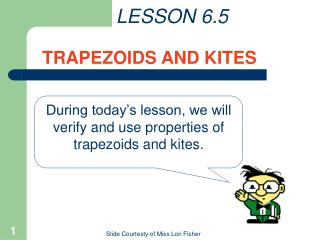DownloadDownload PresentationLESSON 6.5 TRAPEZOIDS AND KITES

# LESSON 6.5 TRAPEZOIDS AND KITES

Download Presentation## LESSON 6.5 TRAPEZOIDS AND KITES

- - - - - - - - - - - - - - - - - - - - - - - - - - - E N D - - - - - - - - - - - - - - - - - - - - - - - - - - -
##### Presentation Transcript

1. LESSON 6.5 TRAPEZOIDS AND KITES During today’s lesson, we will verify and use properties of trapezoids and kites. OBJECTIVE: Verify and use properties of trapezoids and kites Slide Courtesty of Miss Lori Fisher

2. Definitions Trapezoids The base angles of a trapezoid are _________________ ______________________________________________ two angles that share a base of the trapezoid. base base angles leg leg base Slide Courtesty of Miss Lori Fisher

3. B A D C Theorems Trapezoids Theorem 6.15 The base angles of an isosceles trapezoid are __________ Theorem 6.16 The diagonals of an isosceles trapezoid are __________ ____  ____ congruent. congruent. AC BD Slide Courtesty of Miss Lori Fisher

4. A B D C Theorems (continued) Kites Theorem 6.17 The diagonals of a kite are _____________ ____  ____ perpendicular. AC BD Slide Courtesty of Miss Lori Fisher

5. EXAMPLE #1 WXYZ is an isosceles trapezoid, and mX = 156. Find the other missing angles. Slide Courtesty of Miss Lori Fisher

6. EXAMPLE #1 SOLUTIONS: WXYZ is an isosceles trapezoid, and mX = 156. Find the other missing angles. bases are parallel in a trapezoid and angles that share a leg are SSI angles mX + mW = 180 b/c ______________________ ______________________ ______________________ 156 + mW = 180 mW = 24 Because the base angles of an isosceles trapezoid are ________, m___ = m___ = ____ and m___ = m___ = ___ Y X congruent 156 Z W 24 Slide Courtesty of Miss Lori Fisher

7. EXAMPLE # 2 : Find m1, m2, and m3 in the kite. Slide Courtesty of Miss Lori Fisher

8. EXAMPLE #2 SOLUTIONS Find m1, m2, and m3 in the kite. RU = RS by definition of a kite and base angles of an isosceles triangle are congruent. m1 = ____ b/c ____________________________ __________________________________________________________________________________ m2 = ____ b/c ____________________________ _________________________________________ m3 = ____ b/c ____________________________ 72 diagonals of a kite are perpendicular. 90 18 ▲ sum = 180. Slide Courtesty of Miss Lori Fisher

9. HOMEWORK ASSIGNMENT Assignment: pg 322 # 2 - 18 even, 20-25 Using a concept map makes this so much easier! Slide Courtesty of Miss Lori Fisher